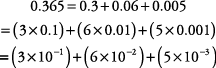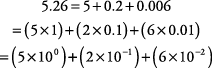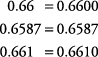## Using the Place Value Grid

The place value grid can be used to assist you in understanding and working with the decimal system.

#### Expanded notation

Decimals can also be written in expanded notation, using the same techniques as when expanding whole numbers.

##### Example 1

Write 0.365 in expanded notation.##### Example 2

Write 5.26 in expanded notation.#### Writing decimals

To read a decimal or write a decimal in words, you start at the left and end with the place value of the last number on the right. Where a whole number is included, use the word “and” to show the position of the decimal point.

##### Example 3

seventy‐five hundredths

##### Example 4

forty‐five and three hundred twenty‐one thousandths

##### Example 5

Write two hundred and three tenths.

200.3

#### Comparing decimals

If you want to compare decimals, that is, find out whether one decimal is greater than another, simply make sure that each decimal goes out to the same number of places to the right.

##### Example 6

Which is greater, 0.37 or 0.365?

0.37 = 0.370, so you can align the two decimals.

0.370

0.365

It is easy to see that 0.37 is greater. You are really comparing three hundred seventy thousandths to three hundred sixty‐five thousandths.

##### Example 7

Put the decimals 0.66, 0.6587, and 0.661 in order from largest to smallest.

First, change each number to ten‐thousandths by adding zeros where appropriate. Then align the decimal points to make the comparison.

0.6600

0.6587

0.6610

The order should be 0.661, 0.66, and 0.6587.

You can also align the decimals first and then add the zeros as follows.Remember: The number of digits to the right of the decimal point does not determine the size of the number (0.5 is greater than 0.33).

#### Rounding decimals

The method for rounding decimals is almost identical to the method used for rounding whole numbers. Follow these steps to round off a decimal:

1. Underline the place value to which you're rounding.

2. Look to the immediate right (one place) of your underlined place value.

3. Identify the number (the one to the right). If it is 5 or higher, round your underlined place value up 1 and drop all the numbers to the right of your underlined number. If the number (the one to the right) is 4 or less, leave your underlined place value as it is and drop all the numbers to the right of your underlined number. (Note that you do not have to replace dropped digits with zeroes.)

##### Example 8

Round off 0.478 to the nearest hundredth.

0.4 78 is rounded up to 0.48.

##### Example 9

Round off 5.3743 to the nearest thousandth.

5.37 43 is rounded down to 5.374